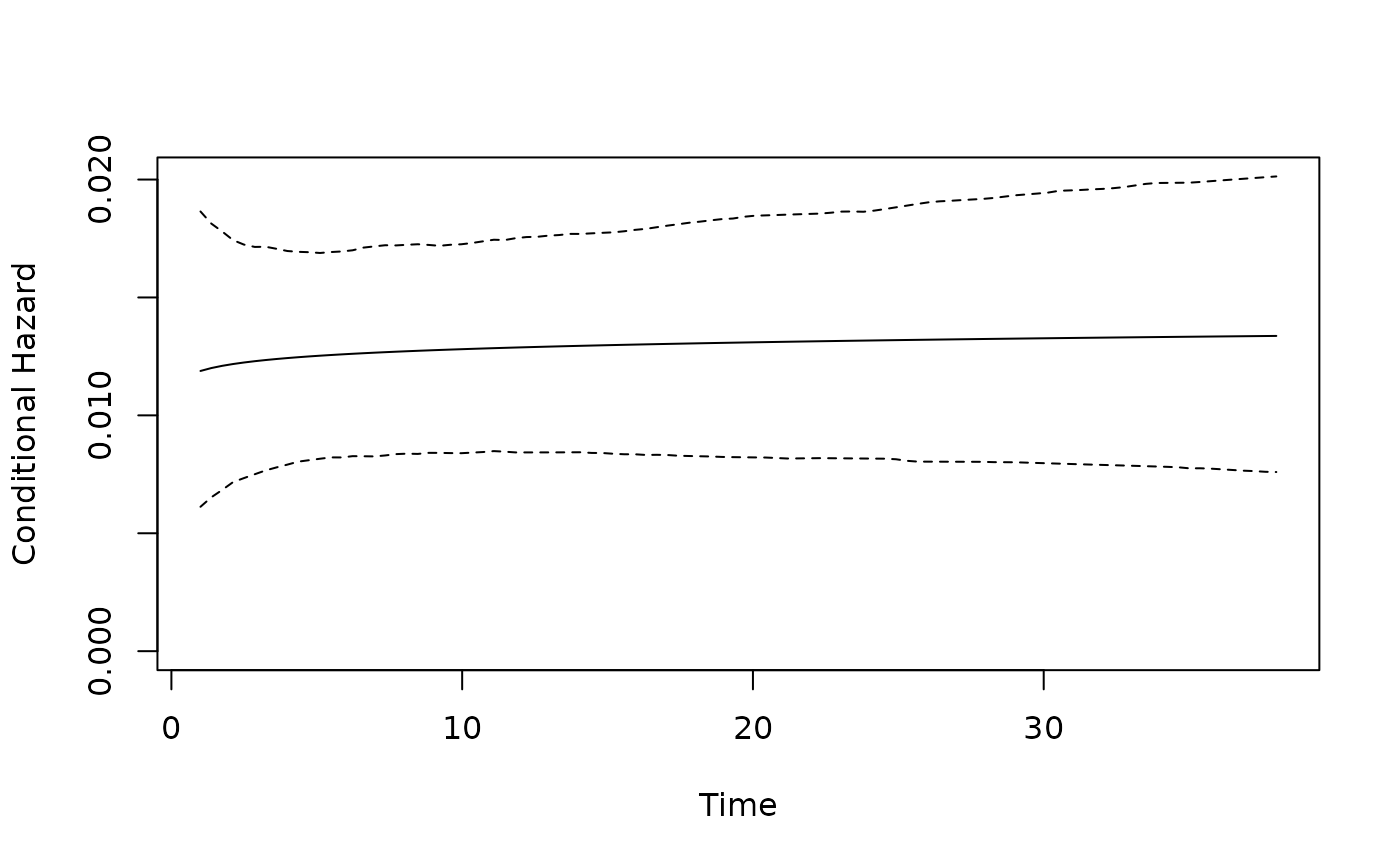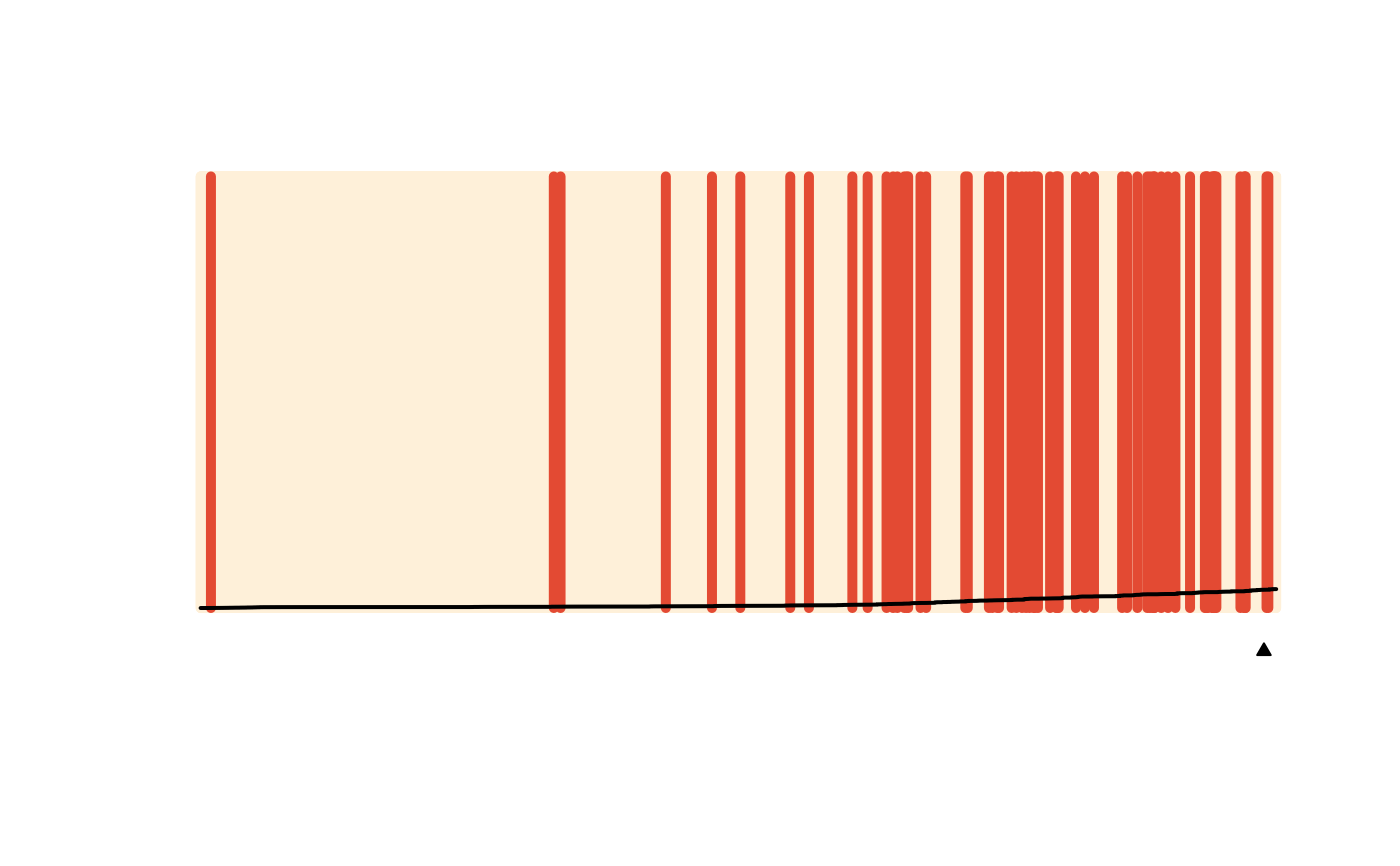Plot results from a spduration model. Two types are currently implemented: a separation plot for evaluating model predictions ("sepplot"), and a plot of the conditional hazard rate ("hazard"), with or without simulation-based confidence intervals.

# S3 method for spdur
plot(x, type = "sepplot", ci = TRUE, ...)

## Arguments

x An object of class "spdur". What kind of plot? "sepplot" or "hazard". For plots of the hazard rate, should a confidence interval be included? Optional parameters passed to sepplot or plot_hazard.

sepplot, plot_hazard
# get model estimates
plot(model.coups, type = "hazard")plot(model.coups)